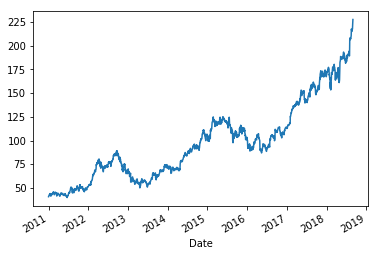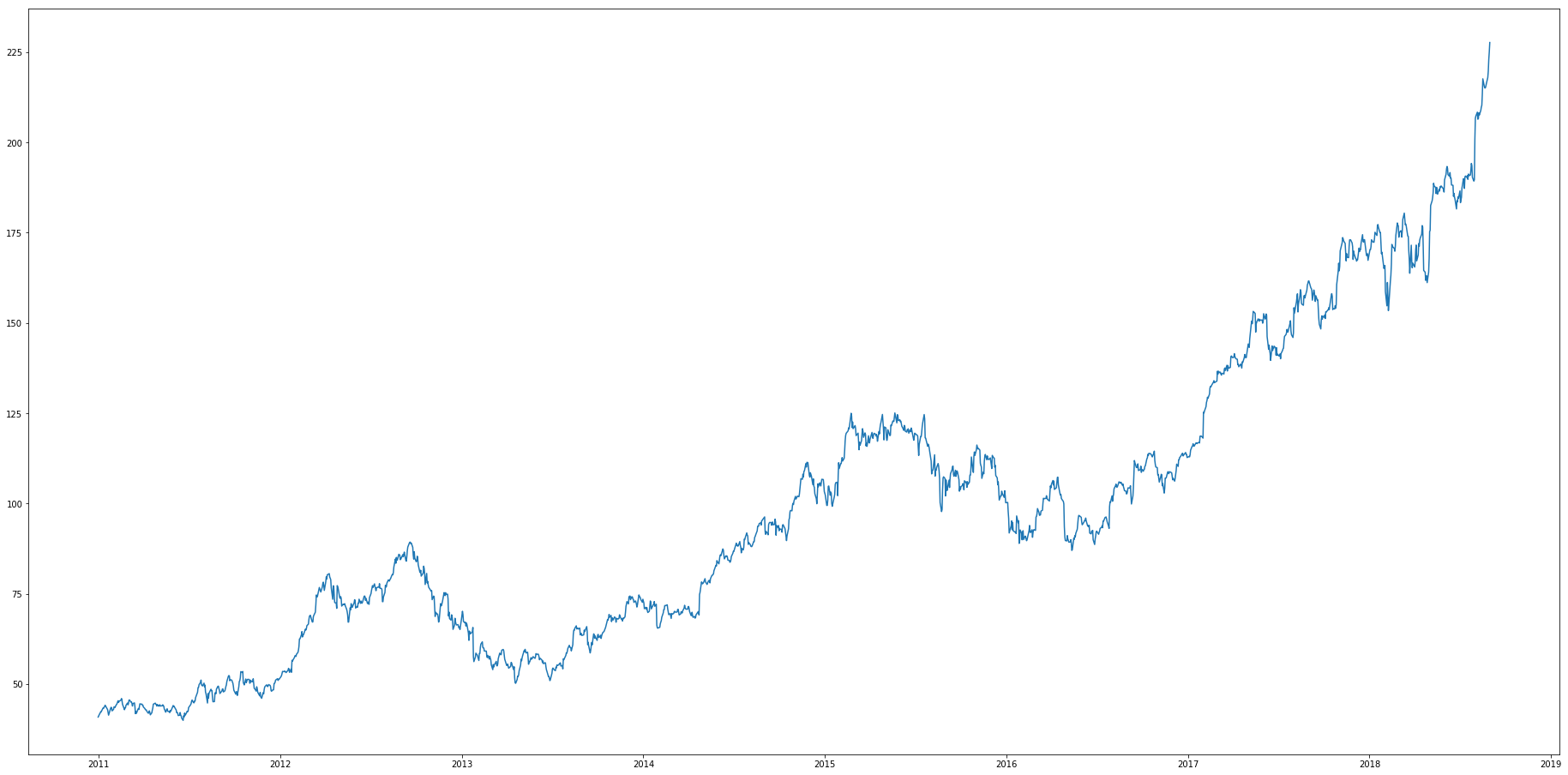# Timeseries forecasting

## Timeseries forecasting¶

Timeseries forecasting can be generally split into two categories

1) Signal processing. Signal processing is typically what is used in engineering and econometrics. ARIMA/GARCH models attempt to filter out the 'signals' from the noise and extrapolate the signals into the future. Famous models for interest rate pricing are 2-factor models (i.e., Vasicek models, Cox-Ingersoll-Ross) models. CIR models allow for mean-reversion,

Vasicek: $dr_t = a(b-r_t)dt+\sigma dW_t$

$a$: speed of reversion
$b$: long-term mean level
$\sigma$: volatility

CIR: $dr_t = a(b-r_t)dt+\sigma \sqrt{r_t} dW_t$
$\sigma \sqrt{r_t}$: removes the possibility of negative interest rates.

2) Curve fitting. Curve fitting is used in models like Facebook's Prophet model, Nelson-Siegel-Svensson models (i.e, term structure of interest rates), spline models. Curve fitting models are very popular in industry.

This post will look at comparing timeseries forecasting models from traditional econometrics vs machine-learning

#### Importing data science modules¶

In :
import numpy as np
import pandas as pd
import matplotlib.pyplot as plt


#### Preprocessing function to normalize data¶

In :
from sklearn.preprocessing import MinMaxScaler
from keras.models import Sequential
from keras.layers import Dense, Dropout, LTSM

scaler = MinMaxScaler(feature_range=(0,1))

Using TensorFlow backend.

---------------------------------------------------------------------------
ImportError                               Traceback (most recent call last)
<ipython-input-34-a124f06fed90> in <module>()
1 from sklearn.preprocessing import MinMaxScaler
2 from keras.models import Sequential
----> 3 from keras.layers import Dense, Dropout, LTSM
4
5 scaler = MinMaxScaler(feature_range=(0,1))

ImportError: cannot import name 'LTSM'

#### Processing stock data¶

1. Read in the data, tell it to parse dates and that dates are the index
In :
df = pd.read_csv('AAPL.csv',parse_dates=,index_col=)

Out:
Open High Low Close Adj Close Volume
Date
2010-12-31 46.135715 46.211430 45.901428 46.080002 40.838676 48377000
2011-01-03 46.520000 47.180000 46.405716 47.081429 41.726177 111284600
2011-01-04 47.491428 47.500000 46.878571 47.327145 41.943951 77270200
2011-01-05 47.078571 47.762856 47.071430 47.714287 42.287071 63879900
2011-01-06 47.817142 47.892857 47.557144 47.675713 42.252876 75107200
In :
df_price = df['Adj Close']
df_price.index

Out:
DatetimeIndex(['2010-12-31', '2011-01-03', '2011-01-04', '2011-01-05',
'2011-01-06', '2011-01-07', '2011-01-10', '2011-01-11',
'2011-01-12', '2011-01-13',
...
'2018-08-20', '2018-08-21', '2018-08-22', '2018-08-23',
'2018-08-24', '2018-08-27', '2018-08-28', '2018-08-29',
'2018-08-30', '2018-08-31'],
dtype='datetime64[ns]', name='Date', length=1931, freq=None)
In :
df_price.plot()

plt.figure(figsize=(32,16))
plt.plot(df_price)

Out:
[<matplotlib.lines.Line2D at 0x26d6588bbe0>]In :
arr_price = df_price.values
train = arr_price[:1500]
test = arr_price[1501:]

In :
arr_price[:5]

Out:
array([40.838676, 41.726177, 41.943951, 42.287071, 42.252876])
In :
train[:5]

Out:
array([40.838676, 41.726177, 41.943951, 42.287071, 42.252876])
In :
test[:5]

Out:
array([112.838768, 113.490685, 113.792313, 113.899338, 113.150139])
In :
### Scaling dataset
scaler = MinMaxScaler(feature_range=(0,1))
scaled_data = scaler.fit_transform(arr_price)

---------------------------------------------------------------------------
ValueError                                Traceback (most recent call last)
<ipython-input-57-2880b9102d5a> in <module>()
1 ### Scaling dataset
2 scaler = MinMaxScaler(feature_range=(0,1))
----> 3 scaled_data = scaler.fit_transform(arr_price)

~\Anaconda3\envs\pelican\lib\site-packages\sklearn\base.py in fit_transform(self, X, y, **fit_params)
460         if y is None:
461             # fit method of arity 1 (unsupervised transformation)
--> 462             return self.fit(X, **fit_params).transform(X)
463         else:
464             # fit method of arity 2 (supervised transformation)

~\Anaconda3\envs\pelican\lib\site-packages\sklearn\preprocessing\data.py in fit(self, X, y)
321         # Reset internal state before fitting
322         self._reset()
--> 323         return self.partial_fit(X, y)
324
325     def partial_fit(self, X, y=None):

~\Anaconda3\envs\pelican\lib\site-packages\sklearn\preprocessing\data.py in partial_fit(self, X, y)
349         X = check_array(X, copy=self.copy, warn_on_dtype=True,
350                         estimator=self, dtype=FLOAT_DTYPES,
--> 351                         force_all_finite="allow-nan")
352
353         data_min = np.nanmin(X, axis=0)

~\Anaconda3\envs\pelican\lib\site-packages\sklearn\utils\validation.py in check_array(array, accept_sparse, accept_large_sparse, dtype, order, copy, force_all_finite, ensure_2d, allow_nd, ensure_min_samples, ensure_min_features, warn_on_dtype, estimator)
545                     "Reshape your data either using array.reshape(-1, 1) if "
546                     "your data has a single feature or array.reshape(1, -1) "
--> 547                     "if it contains a single sample.".format(array))
548
549         # in the future np.flexible dtypes will be handled like object dtypes

ValueError: Expected 2D array, got 1D array instead:
array=[ 40.838676  41.726177  41.943951 ... 222.979996 225.029999 227.630005].
Reshape your data either using array.reshape(-1, 1) if your data has a single feature or array.reshape(1, -1) if it contains a single sample.
In [ ]: